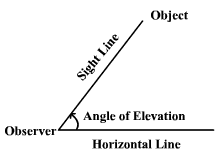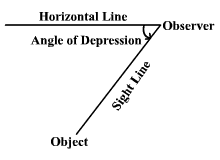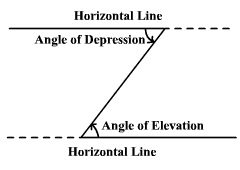# Angles of Elevation & Depression

The term angle of elevation denotes the angle from the horizontal upward to an object.  An observer’s line of sight would be above the horizontal.The term angle of depression denotes the angle from the horizontal downward to an object.  An observer’s line of sight would be below the horizontal.Note that the angle of elevation and the angle of depression are congruent .